# Print Reverse Of A Linked List Without Actually Reversing it

## Introduction to the problem statement

Given a singly-linked list, print it in reverse order without actually reversing it. The only restriction is that you can’t reverse the original linked list.

Let us understand this problem with an example:-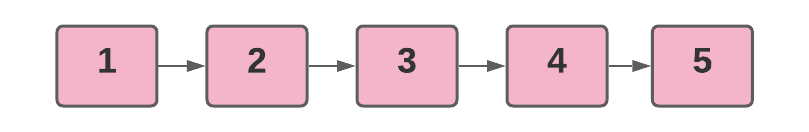Output -

According to the problem, we only need to print it in reverse order.

To specify, you must begin printing the data from the tail and work your way up to the head of the list.

To reverse the list itself, refer to this problem

Before you go further, we recommend you try to implement the problem on your own.

Now that you have understood the problem let us switch to the possible methods to deal with the problem.

## Method 1: Using auxiliary space, stack

As we all know, Stack is a powerful data structure that follows the LIFO( Last In First Out) principle to deal with the data. This signifies that the most recently added element will come out first.Source: DevDojo

Similarly, extracting elements from the stack returns them in the reverse order of insertion. As a result of its LIFO trait, a stack can store elements in reverse order of insertion and hence can be utilised to address our problem.

The idea is to traverse the LinkedList from the head to the tail, pushing each traversed element onto the stack as you go. When the traversal is performed, the data from the linked list will be in reverse order in the stack. We can now extract the top element from the stack, print it, and then pop the elements one at a time, repeating the procedure until the stack is empty.

Now that you've devised a concept, let's look at its algorithm and implementation:

### Algorithm

• Create an empty stack.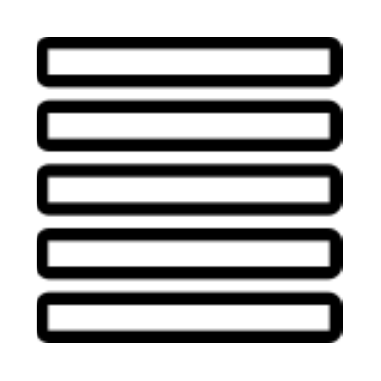• Traverse the linked list and push each node onto the stack one by one.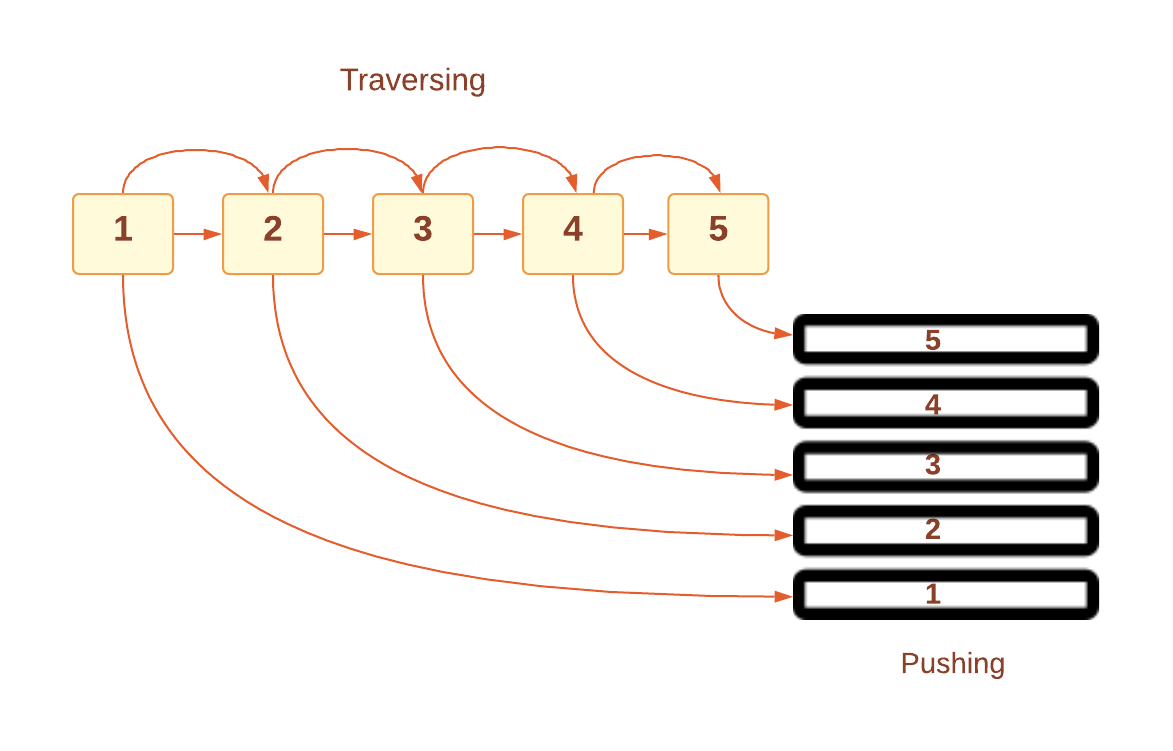• After the traversal is performed, run a loop, in each iteration the topmost element must be printed and then popped out from the stack. Repeat this process until the stack becomes empty.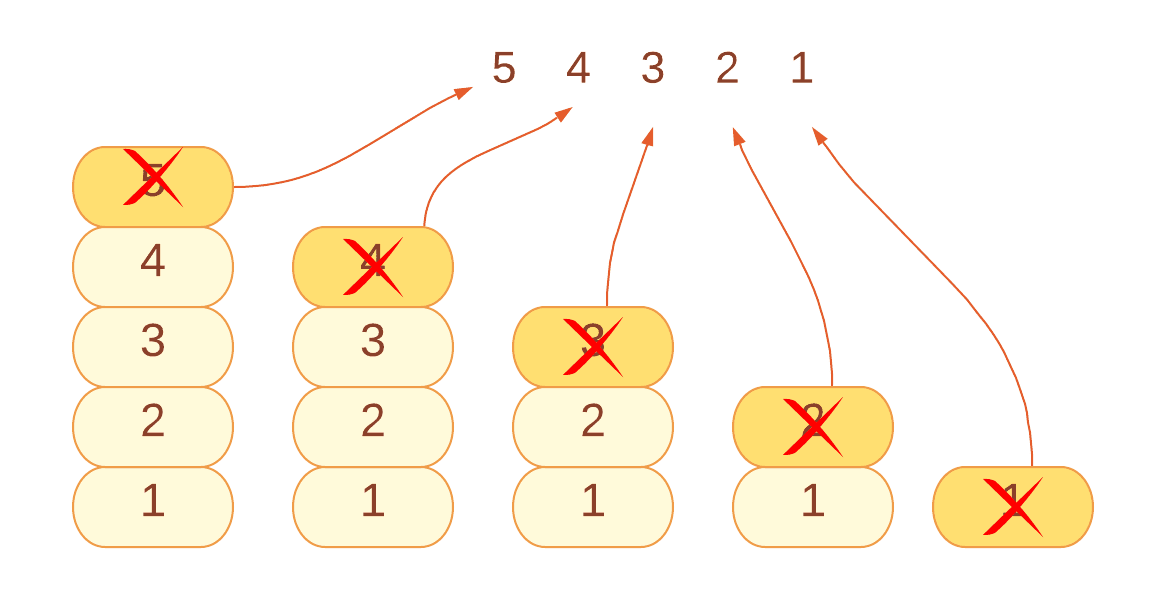As a result, we’ve printed the reverse of a linked list without actually reversing it.

### Implementation in Java

``````// Java program to print reverse of a linked list using stack
// Import all the packages
import java.util.*;
import java.io.*;

// linked list Node with two fields- data, next
class Node
{
int data;
Node next;
Node(int d) {data = d; next = null; }
}

// function to print the reverse
return;
}
// Create an empty stack
Stack<Integer> sc = new Stack<>();
// temp will point to the head
// Run a loop until the temp points to null
while(temp!=null){
// one by one push each temp.data onto the stack
sc.push(temp.data);
// Update the temp to move ahead
temp = temp.next;
}
// Run a loop until the stack becomes empty
while(!sc.empty()){
// get the top most element
int top = sc.peek();
// print the top element
System.out.print(top + "->");
// delete the printed/top element
sc.pop();
}
System.out.println("null");
}
// Utility function to print the linked list
while(temp!=null){
System.out.print(temp.data + "->");
temp = temp.next;
}
System.out.println("null");
}

// Utility function to add the nodes in a list
public void push(int new_data){
Node new_node = new Node(new_data);
}
// main function
public static void main(String args[])
{
// Construct the linked list 1->2->3->4
list.push(4);
list.push(3);
list.push(2);
list.push(1);
// Print the original list
System.out.println("Given list: ");
// print the list in reverse order
System.out.println("Print reverse of a linked list in reverse order: ");
}
}``````

Output

``````Given list:
1->2->3->4->null
Print reverse of a linked list in reverse order:
4->3->2->1->null``````

#### Complexity Analysis

Time Complexity: If we count the no. of operations we’ve performed through the program will be:

1. Traversing of each node - o(N), where N is the number of total nodes in a list.
2. Pushing onto the stack - o(1), constant time.
3. Printing the stack - o(N), where N is the number of pushed nodes onto the stack.

Hence, the time complexity to print reverse of a linked list will be o(N) + o(N) +  o(1) =  o(2N+1) ~ O(N), where N is the total number of nodes in the list.

Space Complexity: Since we are utilising an additional data structure stack for storing the nodes, space complexity would be O(n), where n is the total number of pushed nodes, i.e, the total nodes in a given linked list.

The approach we’ve discussed above is not optimized in the context of space.

Can you think of a better approach for this?

There is another approach that may not give us a better time complexity yet relieve us not to use auxiliary space.

Let us discuss now:

## Method 2: Recursion

The idea is to traverse the linked list recursively. Since recursion preserves a stack of calls during the execution, we would have a choice to print the nodes in reverse order.

Let's have a look at the algorithm for this now:

### Algorithm

• Make a function printReverse(), that will accept the head of the list as a parameter.
• Base case: If the linked list is empty, i.e., the head is null, simply return from the function.
• Make a recursive call by passing the next node of a list as an argument.
• Print the node.data.

We will have our linked list in reverse order.

Consider the below representation to understand the algorithm: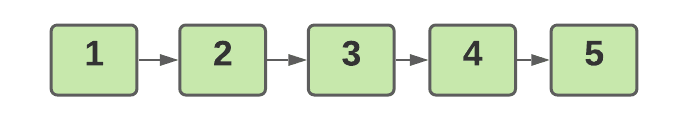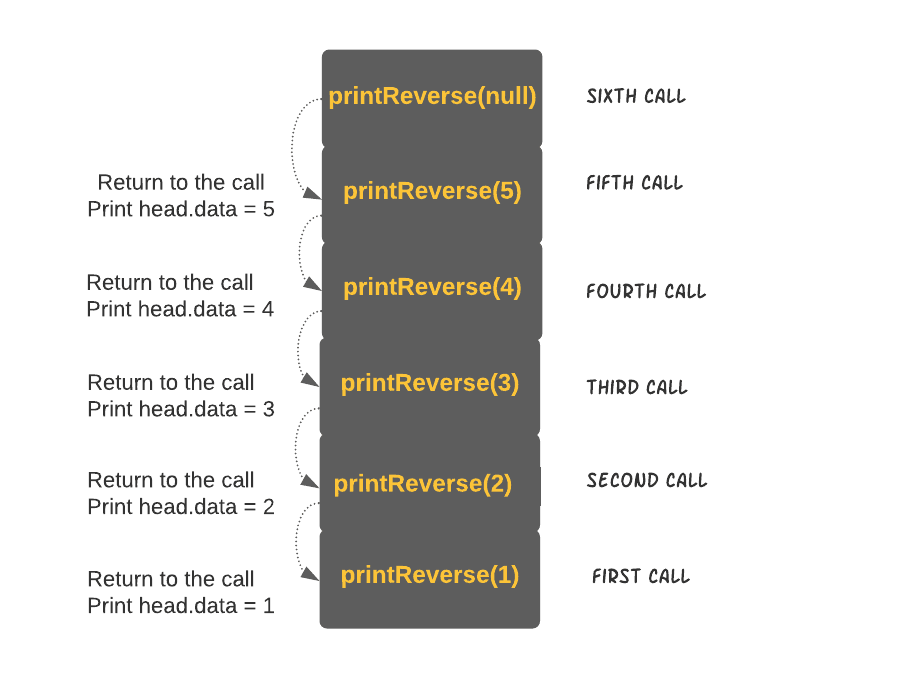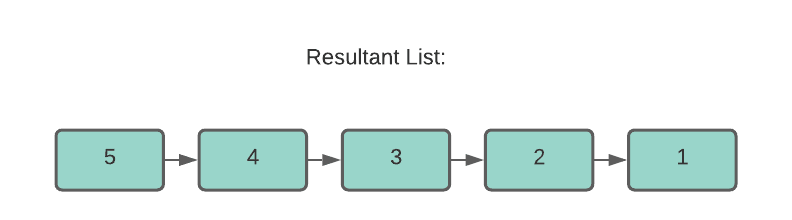### Implementation in java

``````// Java program to print reverse of a linked list using recursion
// Import all the packages
import java.util.*;
import java.io.*;

// linked list Node with two fields- data, next
class Node
{
int data;
Node next;
Node(int d) {data = d; next = null; }
}

// function to print the reverse
return;
}
// make a recursive call the next node
// print the node
}
// Utility function to print the linked list
while(temp!=null){
System.out.print(temp.data + " ");
temp = temp.next;
}
}

// Utility function to add the nodes in a list
public void push(int new_data){
Node new_node = new Node(new_data);
}
// main function
public static void main(String args[])
{
// Construct the linked list 1->2->3->4
list.push(4);
list.push(3);
list.push(2);
list.push(1);
// Print the original list
System.out.println("Given list: ");
// print the list in reverse order
System.out.println("\nPrint reverse of a linked list in reverse order: ");
}
}``````

Output

``````Given list:
1 2 3 4
Print reverse of a linked list in reverse order:
4 3 2 1 ``````

#### Complexity Analysis

Time Complexity: Since we are traversing each node, the time taken to complete the traversal would be O(N), where N is the total number of nodes in a list.

Space Complexity: Since we’ve employed recursion which maintains a stack for each call, the space would be O(N), where N is the total number of recursive calls.

1. What is the time complexity of traversal operation?
The traversal operation visits each member of the data structure once, implying that the larger the number of elements/nodes, the longer it takes to traverse.
For example, suppose you have two linked lists, one with five nodes and the other with ten. It will take less time to go through the first list than it would to go through the second, i.e., O(5) < O(10).
2. How is the linked list represented in memory?
1Array-based static representation: A static representation of a single linked list maintains two arrays: data and links.
2Free pool of storage dynamic representation: A free storage pool is a collection of free memory spaces. When a node is needed during the creation of a linked list, the program sends a request to the memory to allocate the memory block.
3. Why is a linked list stored in heap memory?
The memory of a computer is split into heap and stack segments. The stack is smaller than the heap and has a set size.
On the other hand, the linked list has the main advantage of dynamically extending itself as needed without worrying about memory usage. As a result, a heap is an optimal location for storing linked list objects.

## Key takeaways

To cover up the session, we’ve looked at two approaches for printing a Linked List without actually reversing it. Approaches are many, but which are more optimized is needed. This was a fundamental question yet important for logic building and finalizing which method is more optimal.

Similar problems you can look upon:

Furthermore, have a look at our top-notch courses just for you to have a bright and promising future.

Keep coding and booming, Ninja!